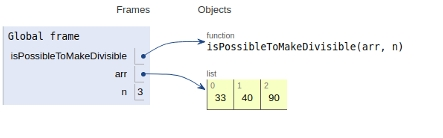# Python Program to check whether it is possible to make a divisible by 3 number using all digits in an array

In this article, we will learn about the solution and approach to solve the given problem statement.

## Problem statement

Given an array input of integers, we need to find whether it’s possible to make an integer using all the digits available in these numbers such that it would be divisible by 3.

Here we will generate a function that will take two arguments namely the array of integers and the length of the array.

The implementation given below works on the concept from the mental mathematics. Here we observe that a number is divisible by 3 if the sum of the digits are divisible by 3.

Now let’s see the implementation below −

## Example

Live Demo

def isPossibleToMakeDivisible(arr, n):
remainder = 0
for i in range (0, n):
remainder = (remainder + arr[i]) % 3
return (remainder == 0)
# main()
arr = [33,40,90]
n = 3
if (isPossibleToMakeDivisible(arr, n)):
print("Yes")
else:
print("No")

## Output

No

All variables and functions are declared in global scope as shown in the figure below.## Conclusion

In this article, we learnt about the approach to find whether it is possible to make a divisible by 3 number using all digits in an array.